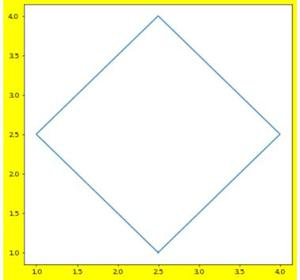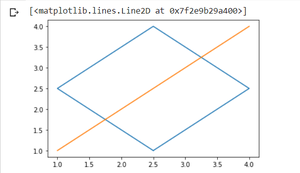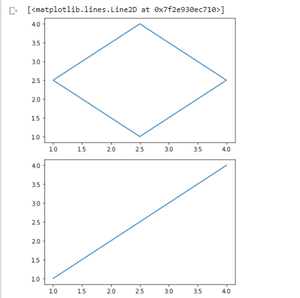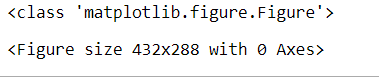Related Articles

# How to modify existing figure instance in Matplotlib?

• Last Updated : 04 Sep, 2021

In Python matplotlib.pyplot.figure() is used to modify existing Figure instance or make a new Figure instance. Generally, it is used to alter the basic properties of existing plots. It takes references to the concerned plots in case if it is used to alter the properties of the already formed plots. It returns the Figure instance and passes the same to new_figure_manager in the backend, which in return allows to custom hook Figure classes into the pyplot interface.

Syntax: matplotlib.pyplot.figure(num=None, figsize=None, dpi=None, facecolor=None, edgecolor=None,
frameon=True, FigureClass=<class ‘matplotlib.figure.Figure’>, clear=False, **kwargs)

Parameters

num : It is a unique identifier for the figure. If the Figure instance is already there with the same value, that instance is returned else a new instance is created and random number assigned to it. In case the value is number then it acts as a unique id and in case of string it also acts as Image label. This parameter is optional.

figsize: It is a parameter which takes in a tuple or a list of 2 floats mentioning the width and height of the figure respectively. By default it is set as [6.4, 4.8]

dpi: As the name suggest, it takes in the dots per inch as a float value. Default value is 100.

facecolor : It is used to set the background color of the figure. Default value is ‘white’.

edgecolor: It is used to set the border color of the figure. Default value is ‘white’.

FigureClass: Takes in the subclass of Figure Optionally used for referring any custom Figure instance.

clear: used to clear any existing figure on the respective instance.

Return: instance.close()

It is recommended to use the .close() method after using figure to use a clean the unnecessary memory. so to close the figure instance, write.

Example 1: Extract a Figure instance using a figure()

The example mentioned below shows how the figure method is used to label any plot and using this same label as a reference point to fetch the Figure instance. The asset statement confirms the fact that both instances are pointing to the same reference point.

## Python3

 `import` `matplotlib.pyplot as plt` `# Figure instance with label => lebel``fig ``=` `plt.figure( num ``=` `'label'` `)` `fig.get_label()` `# This will fetch Figure instance fig only``fig2 ``=` `plt.figure( num ``=` `'label'` `)``assert` `fig ``=``=` `fig2`

Example 2: Plotting graph with custom height, width, and background

This example tells about how to plot a graph using the custom size of any plot and using custom dpi along with it. Here the background is also changed to yellow from white. The height is set to 10 and width to 7.

## Python3

 `import` `matplotlib.pyplot as plt` `# plotting a yellow background``# graph with dpi => 50``plt.figure(num``=``'label'``,``           ``facecolor``=``'yellow'``,``           ``figsize``=``[``10``, ``7``],``           ``dpi``=``50``)` `plt.plot([``2.5``, ``1``, ``2.5``, ``4``, ``2.5``],``         ``[``1``, ``2.5``, ``4``, ``2.5``, ``1``])`

OutputYellow background

Example 3: Example to clear the graph

The first code here is to show how the code will if two different plots are made on a single instance without using clear. The ideal thing that will work here is that it will plot both of them on a single figure.

## Python3

 `import` `matplotlib.pyplot as plt` `plt.plot([``2.5``, ``1``, ``2.5``, ``4``, ``2.5``],``         ``[``1``, ``2.5``, ``4``, ``2.5``, ``1``])` `plt.plot([``1``, ``2``, ``3``, ``4``], [``1``, ``2``, ``3``, ``4``])`

OutputBoth plotted

Now execute the same code but by clearing the first plot just before implementing the 2nd one.

## Python3

 `import` `matplotlib.pyplot as plt` `plt.plot([``2.5``, ``1``, ``2.5``, ``4``, ``2.5``],``         ``[``1``, ``2.5``, ``4``, ``2.5``, ``1``])` `# This will clear the first plot``plt.figure(clear``=``True``)` `# This will make a new plot on a``# different instance``plt.plot([``1``, ``2``, ``3``, ``4``], [``1``, ``2``, ``3``, ``4``])`

OutputTwo different plots on clearing

Example 4: Check the return type

The figure() returns a Figure instance, the next example just verifies this fact.

## Python3

 `import` `matplotlib.pyplot as plt` `# the type comes out as Figure Instance.``print``(``type``(plt.figure()))`

Output:Attention geek! Strengthen your foundations with the Python Programming Foundation Course and learn the basics.

To begin with, your interview preparations Enhance your Data Structures concepts with the Python DS Course. And to begin with your Machine Learning Journey, join the Machine Learning – Basic Level Course

My Personal Notes arrow_drop_up